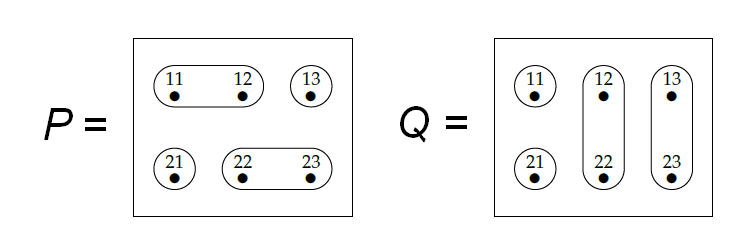Okay, Charlie: here's what I _should_ have said, and now do:

To prove that sometimes

$f^{\ast}(P \vee Q) \ne f^{\ast}(P) \vee f^{\ast}(Q) ,$

we just need one example. So, take \$$P\$$ and \$$Q\$$ to be these two partitions:They are partitions of the set

$Y = \\{11, 12, 13, 21, 22, 23 \\}.$

Take \$$X = \\{11,22\\} \$$ and let \$$i : X \to Y \$$ be the inclusion of \$$X\$$ into \$$Y\$$, meaning that

$i(11) = 11, \quad i(22) = 22 .$

Then compute everything! \$$11\$$ and \$$22\$$ are in different parts of \$$i^{\ast}(P)\$$:

$i^{\ast}(P) = \\{ \\{11\\}, \\{22\\} \\} .$

They're also in different parts of \$$i^{\ast}(Q)\$$:

$i^{\ast}(Q) = \\{ \\{11\\}, \\{22\\} \\} .$

Thus, we have

$i^{\ast}(P) \vee i^{\ast}(Q) = \\{ \\{11\\}, \\{22\\} \\} .$

On the other hand, the join \$$P \vee Q \$$ has just two parts:

$P \vee Q = \\{\\{11,12,13,22,23\\},\\{21\\}\\} .$

If you don't see why, figure out the finest partition that's coarser than \$$P\$$ and \$$Q\$$ - that's \$$P \vee Q \$$. Since \$$11\$$ and \$$22\$$ are in the same parts here, the pullback \$$i^{\ast} (P \vee Q) \$$ has just one part:

$i^{\ast}(P \vee Q) = \\{ \\{11, 22 \\} \\} .$

So, we have

$i^{\ast}(P \vee Q) \ne i^{\ast}(P) \vee i^{\ast}(Q)$

as desired.